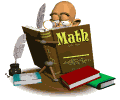•Numbers to 10,000
Mental Math and Estimation
Addition and Subtraction up to 10,000
Multiplication and Division
Using Bar Models: Multiplication and Division
Metric Length, Mass, and Volume & Real-World Problems
Bar Graphs and Line Plots
Two-Dimensional Shapes
Area and Perimeter
Fractions